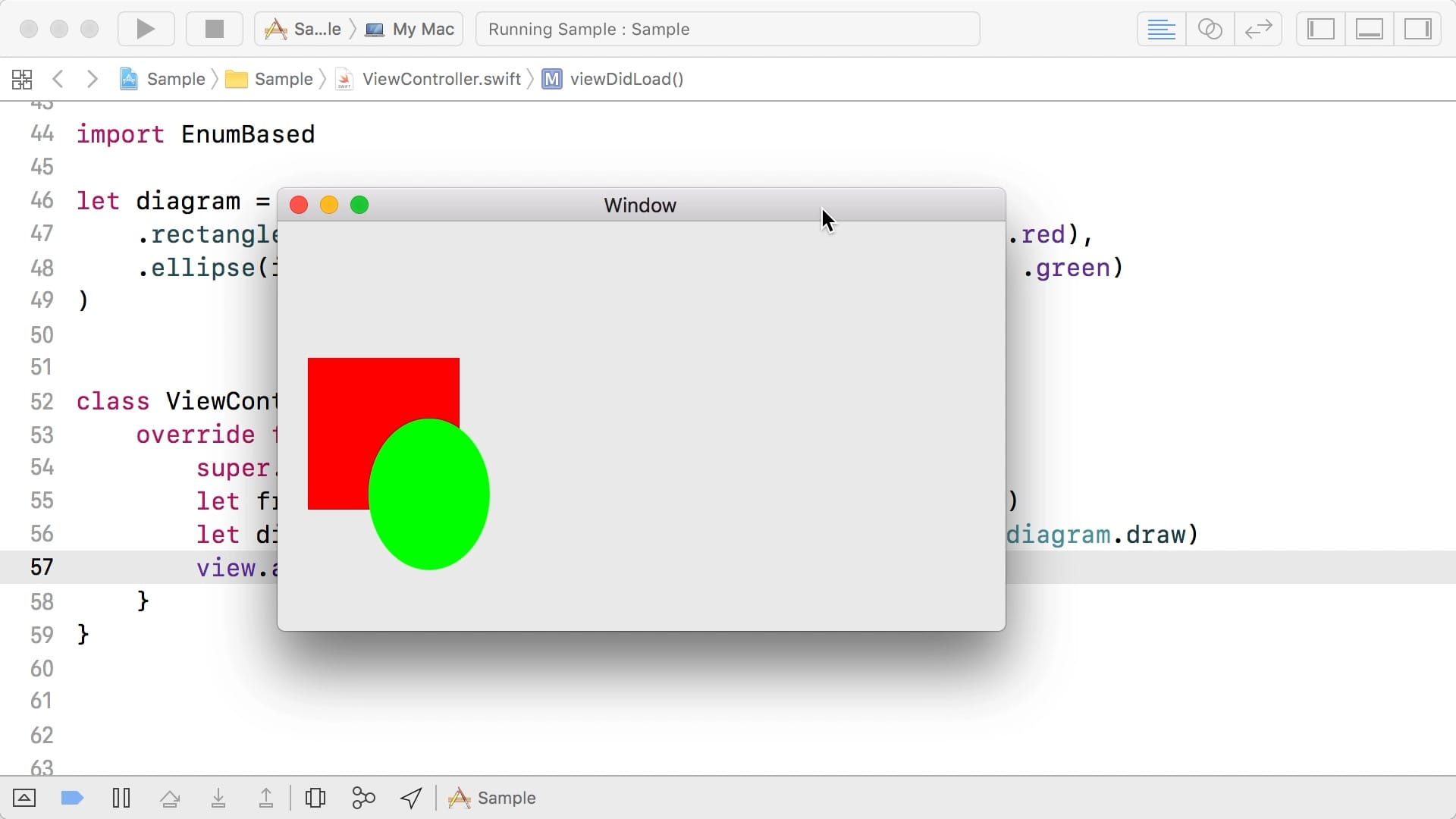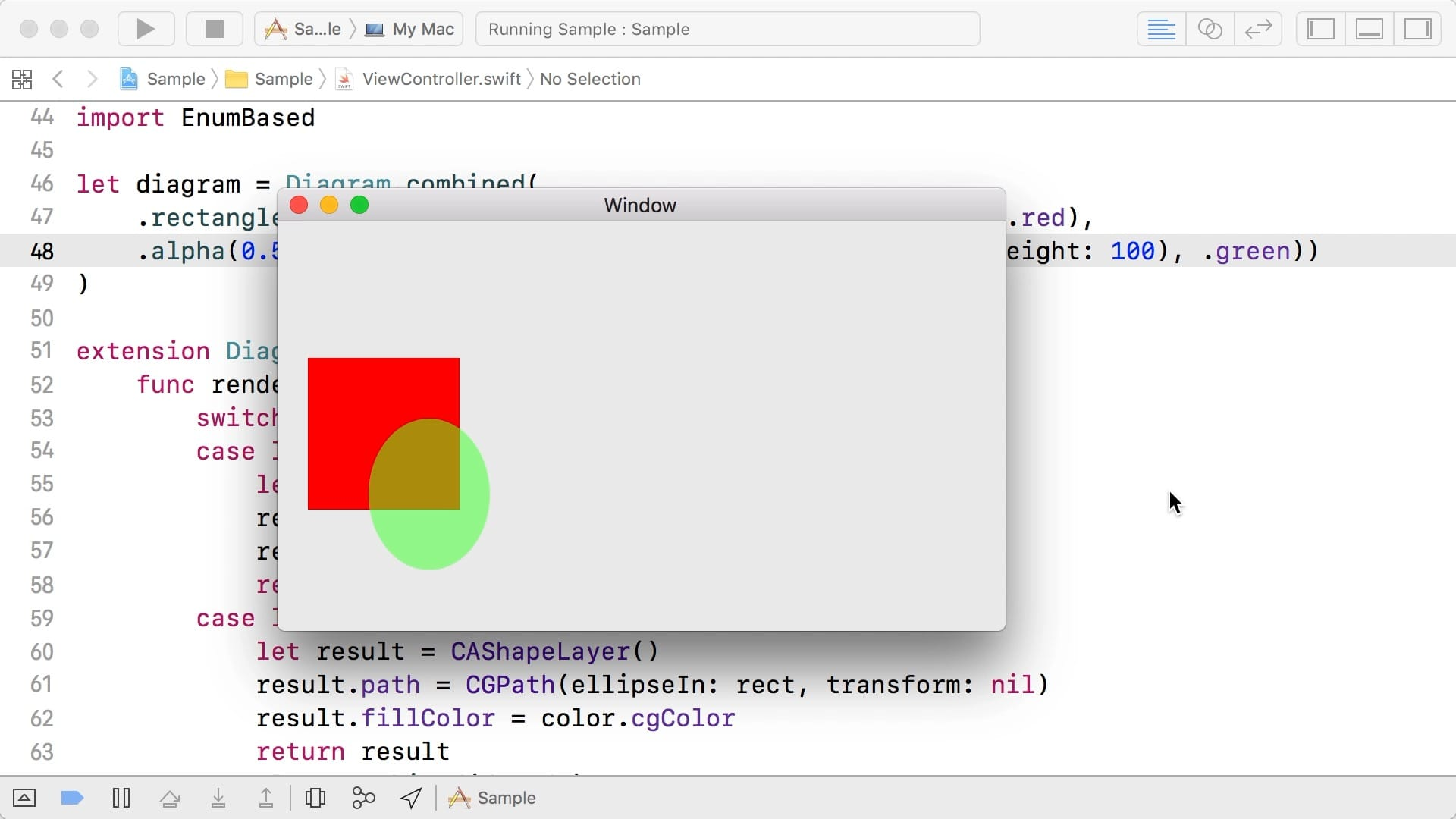Swift Talk # 88

## Extensible Libraries 1: Enums vs Classes

with special guest Brandon Kase

### This episode is freely available thanks to the support of our subscribers

We discuss the capabilities and limitations of enums and classes when designing extensible libraries.

00:06 Brandon from Pinterest joins us again today. Last time he was on, we discussed phantom types. This time, we'll talk about extensible libraries.

00:42 We've prepared two versions of a diagrams library. The first version is called `EnumBased`, and this is its public interface:

``````public enum Diagram {
case rectangle(CGRect, NSColor)
case ellipse(in: CGRect, NSColor)
case combined(EnumBased.Diagram, EnumBased.Diagram)
}

extension Diagram {
public func draw(_ context: CGContext)
}
``````

01:07 The library defines enum-based diagrams. A diagram can be a rectangle or an ellipse, or a recursive combination of diagrams. And a diagram can render itself into a `CGContext`.

01:40 Let's try using the library and create a diagram:

``````import EnumBased

let diagram = Diagram.combined(
.rectangle(CGRect(x: 20, y: 20, width: 100, height: 100), .red),
.ellipse(in: CGRect(x: 60, y: 60, width: 80, height: 100), .green),
)
``````

02:57 We prepared a view subclass, `CGContextView`, that draws itself by calling a render closure with its current graphics context. To make this diagram show up in a sample Mac app, we create one of these views and pass in our diagram's `draw` method as the render closure:

``````class ViewController: NSViewController {
let frame = CGRect(x: 0, y: 0, width: 200, height: 200)
let diagramView = CGContextView(frame: frame, render: diagram.draw)
}
}
``````## Extending an Enum-Based Library

04:03 Now that everything's in place, we want to try extending the `EnumBased` library. Let's say that, instead of using the `CGContext` renderer, we want to render using `CALayer`. We add an extension for `Diagram` with a method that produces a `CALayer`:

``````extension Diagram {
func render() -> CALayer {
switch self {
case let .rectangle(rect, color):
let result = CALayer()
result.frame = rect
result.backgroundColor = color.cgColor
return result
case let .ellipse(rect, color):
let result = CAShapeLayer()
result.path = CGPath(ellipseIn: rect, transform: nil)
result.fillColor = color.cgColor
return result
case let .combined(d1, d2):
let result = CALayer()
return result
}
}
}
``````

07:21 We update the view controller to call the new render method. We use another view subclass, `LayerView`, which takes a custom `CALayer` and sets it as its layer:

``````class ViewController: NSViewController {
let frame = CGRect(x: 0, y: 0, width: 200, height: 200)
let diagramView = LayerView(frame, diagram.render())
}
}
``````

08:02 The diagram looks exactly the same as before, but this time, it's rendered with layers. So far so good, but now we want to extend the library in another dimension: by adding a new type of diagram. Specifically, we want to add a primitive that changes the opacity of another diagram.

08:37 Since `Diagram` is defined as an enum and we want to add a new case, we have to make changes to the library itself. If we were dealing with a library from CocoaPods or Carthage, we would have to fork the library. But in this case, we control the library, so we can open it and add the `.alpha` case to the enum:

``````public enum Diagram {
case rectangle(CGRect, NSColor)
case ellipse(in: CGRect, NSColor)
indirect case combined(Diagram, Diagram)
indirect case alpha(CGFloat, Diagram)
}
``````

09:29 The compiler immediately tells us about any switches that are no longer exhaustive. First, we have to deal with the new `.alpha` case in the `draw` method:

``````extension Diagram {
public func draw(_ context: CGContext) {
context.saveGState()
switch self {
case let .rectangle(rect, color):
context.setFillColor(color.cgColor)
context.fill(rect)
case let .ellipse(rect, color):
context.setFillColor(color.cgColor)
context.fillEllipse(in: rect)
case let .combined(d1, d2):
d1.draw(context)
d2.draw(context)
case let .alpha(alpha, d):
context.setAlpha(alpha)
d.draw(context)
}
context.restoreGState()
}
}
``````

10:37 That fixes the context renderer, but we also have to fix the layer render method, which we added in our own code:

``````extension Diagram {
func render() -> CALayer {
switch self {
// ...
case let .alpha(alpha, d):
let result = CALayer()
result.opacity = Float(alpha)
return result
}
}
}
``````

11:36 Now we can use an alpha primitive in our diagram:

``````let diagram = Diagram.combined(
.rectangle(CGRect(x: 20, y: 20, width: 100, height: 100), .red),
.alpha(0.5, .ellipse(in: CGRect(x: 60, y: 60, width: 80, height: 100), .green))
)
``````12:04 We extended the `EnumBased` library in two ways. Adding a method with an extension was easy, but to add a new primitive, we had to go into the library and edit its source code.

## Extending a Class-Based Library

12:23 We also prepared a class-based version of the diagram library. Its interface looks like this:

``````open class Diagram {
public init()
open func draw(_ context: CGContext)
}

public class Rectangle : Diagram {
public init(_ rect: CGRect, _ color: NSColor)
override public func draw(_ context: CGContext)
}

public class Ellipse : Diagram {
public init(in rect: CGRect, _ color: NSColor)
override public func draw(_ context: CGContext)
}

public class Combined : Diagram {
public init(_ d1: Diagram, _ d2: Diagram)
override public func draw(_ context: CGContext)
}
``````

13:02 We now have a base class, `Diagram`, and it exposes a `draw` method. All specific diagrams are subclasses, each with their own initializer and an override of the `draw` method.

13:28 We can rewrite our sample diagram in terms of this class-based library:

``````let diagram = Combined(
Rectangle(CGRect(x: 20, y: 20, width: 100, height: 100), .red),
Ellipse(in: CGRect(x: 60, y: 60, width: 80, height: 100), .green)
)
``````

14:44 In the view controller, we switch back to using the `CGContextView`. The rest of the code is identical to what we had before, and it results in the same shape rendered in our app:

``````class ViewController: NSViewController {
let frame = CGRect(x: 0, y: 0, width: 200, height: 200)
let diagramView = CGContextView(frame: frame, render: diagram.draw)
}
}
``````

15:00 We also want to extend this library, starting with an alpha primitive. Since `Diagram` is defined as a class, we can simply add our own subclass. We don't need to go into the library to add it; we can write the subclass in our own code:

``````class Alpha: Diagram {
let alpha: CGFloat
let diagram: Diagram
init(alpha: CGFloat, diagram: Diagram) {
self.alpha = alpha
self.diagram = diagram
}
}

let diagram = Combined(
Rectangle(CGRect(x: 20, y: 20, width: 100, height: 100), .red),
Alpha(alpha: 0.5, diagram: Ellipse(in: CGRect(x: 60, y: 60, width: 80, height: 100), .green))
)
``````

16:47 After we add an alpha primitive to our diagram and we run the app, the ellipse is gone, because we forgot to override the draw method in `Alpha` (and the compiler didn't remind us to do so):

``````class Alpha: Diagram {
// ...
override func draw(_ context: CGContext) {
context.saveGState()
context.setAlpha(alpha)
diagram.draw(context)
context.restoreGState()
}
}
``````

18:01 The ellipse is now drawn with the opacity we want. And it was pretty easy to add the `Alpha` subclass — we just had to be careful and remember to override `draw`.

18:17 Let's think about how to approach the other library extension: adding the `CALayer` render method to `Diagram`. Drawing is done in the base class, so if we want to add another drawing method, we have to write it in the base class. If the base class is defined in a library, we, as mere consumers of the library, are stuck again. The only viable solution is to go into the source code of the library and add the new method there.

## The Expression Problem

19:37 It's very easy to extend the enum-based library when we only want to add an interpretation, like the new render method. But to add a new primitive, we have to fork the library. With the class-based library, we have the opposite problem. It's easy to add a primitive, but we have to fork the library to add a new render method. When we write a library, we have to carefully consider these tradeoffs — especially if we release the library for others to consume.

20:47 The class-based approach is exactly how UIKit works. The library defines `UIView` and we can subclass it, but we can't change what a view is or, for example, how it renders. If we want to take an existing view hierarchy and, say, make it `Codable`, it's impossible without going into UIKit and modifying it — which we can't do because we don't have access to the source code.

21:28 The challenges we faced today are described by what's commonly referred to as the expression problem: it's really hard to write a library, to which, from the consumer's side, we can add both new items and new interpretations, without forking the library, and while maintaining type safety. But there is hope: we'll see a neat way to solve this problem in the next episode.

### Resources

• #### Sample Project

Written in Swift 4

### Related Blogposts

• #### Swift Tip: Enums vs. Protocols

May 06, 2019

Extensible in different ways

• #### Swift Tip: Enums vs Classes

February 19, 2018

The Expression Problem

See All
Experiments

### Git Under the Hood: Trees

Episode 358 · Jun 02

Experiments

### Git Under the Hood: Blobs

Episode 357 · May 26

SwiftUI

### Connecting Lines with Anchors (Part 3)

Episode 356 · May 19

SwiftUI

### Connecting Lines with Anchors (Part 2)

Episode 355 · May 12

SwiftUI

### Connecting Lines with Anchors (Part 1)

Episode 354 · May 05

SwiftUI

### Reimplementing Anchors: Transforms

Episode 353 · Apr 28

SwiftUI

### Reimplementing Anchors: Points

Episode 352 · Apr 21

SwiftUI

### Reimplementing Anchors: Bounds

Episode 351 · Apr 14

Unlock Full Access

## Subscribe to Swift Talk

• ### Watch All Episodes

A new episode every week